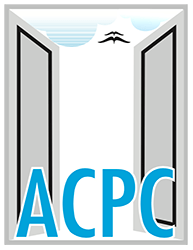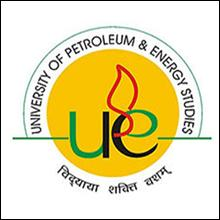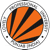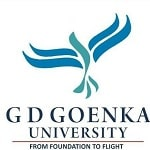# Gujarat PGCET 2022 Syllabus – Check Subject & Section Wise SyllabusGujarat PGCET is completely known as Gujarat Post Graduate Common Entrance Test and it is the state level entrance examination, which is organized through the Admission Committee for Professional Courses (ACPC) to offer admission to the applicants in the first year of the Professional Post-Graduate Courses.

This examination is conducted to offer admission to the applicants in various professional courses in M.Tech/M.E. to various Institutes in the state of Gujarat.

Those students want to get admission in these courses from the colleges of Gujarat State; they can apply in Gujarat.

## Details of PGCET Syllabus

 Name of Discipline Discipline Code Civil Engineering CE Mechanical Engineering ME Electrical Engineering EE Electronics & Communication EC Instrumentation & Control IC Engineering IC Chemical Engineering CH Environment Engineering EN Metallurgy MT Textile Engineering TE Computer Engineering and Information Technology CO Bio-Medical Engineering BM Mechatronics Engineering MC Pharmacy PH Networking, Communication & Web Technology NW

## Civil Engineering (CE)

Latest Applications For Various UG & PG Courses Open 2022:

1. UPES, Dehradun | Admissions Open for All Courses 2022. Apply Now
2. Lovely Professional University, Punjab | 2022 Admissions Open for All Courses. Apply Now
3. Chandigarh University | Admissions Open for All Courses 2022. Apply Now
4. DIT University, Dehradun | Admissions Open for All Courses 2022. Apply Now
5. MIT WPU, Pune | Admissions Open for All Courses 2022. Apply Now
6. Parul University, Vadodara | UG & PG Admission Open 2022. Apply Now
7. Bennett University, Greater Noida | Admission Open at Bennett University 2022. Apply Now
8. Sharda University, Greater Noida |  Admission Open at Sharda University 2022. Apply Now
9. GD Goenka University, Gurugram | UG & PG Admission Open 2022. Apply NowEngineering Mathematics

Linear Algebra: Matrix algebra, Systems of linear equations, Eigenvalues, and eigenvectors.

Calculus: Functions of a single variable, Limit, continuity and differentiability, Mean value theorems, Evaluation of definite and improper integrals, Partial derivatives, Total derivative, Maxima and minima, Gradient, Divergence and Curl, Vector identities, Directional derivatives, Line, Surface and Volume integrals, Stokes, Gauss and Green’s theorems.

Differential equations: First order equations (linear and nonlinear), Higher order linear differential equations with constant coefficients, Cauchy’s and Euler’s equations, Initial and boundary value problems, Laplace transforms, Solutions of one-dimensional heat and wave equations, and Laplace equation.
Complex variables: Analytic functions, Cauchy’s integral theorem, Taylor and Laurent series.

Probability and Statistics: Definitions of probability and sampling theorems, Conditional probability, Mean, median, mode and standard deviation, Random variables, Poisson, Normal and Binomial distributions.

Numerical Methods: Numerical solutions of linear and non -linear algebraic equations
Integration by trapezoidal and Simpson’s rule, single and multi-step methods for differential equations.

## Structural Engineering

Mechanics: Bending moment and shear force in statically determinate beams.

Simple stress and strain relationship: Stress and strain in two dimensions, principal stresses, stress transformation, Mohr’s circle.
Simple bending theory, flexural and shear stresses, unsymmetrical bending, shear center. Thin-walled pressure vessels, uniform torsion, buckling of column, combined and direct bending stresses.

Structural Analysis: Analysis of statically determinate trusses, arches, beams, cables, and frames, displacements in statically determinate structures and analysis of statically indeterminate structures by force/ energy methods, analysis by displacement methods (slope deflection and moment distribution methods), influence lines for determinate and indeterminate structures. Basic concepts of matrix methods of structural analysis.

Concrete Structures: Concrete Technology- properties of concrete, basics of mix design. Concrete design basic working stress and limit state design concepts, analysis of ultimate load capacity, and design of members subjected to flexure, shear, compression, and torsion by limit state methods. Basic elements of prestressed concrete, analysis of beam sections at transfer, and service loads.

Steel Structures: Analysis and design of tension and compression members, beams and beam-columns, column bases. Connections- simple and eccentric, beam-column connections, plate girders, and trusses. Plastic analysis of beams.

## Geotechnical Engineering

Soil Mechanics: Origin of soils, soil classification, three-phase system, fundamental definitions, relationship and interrelationships, permeability & seepage, effective stress principle, consolidation, compaction, shear strength.

Foundation Engineering: Sub-surface investigations- scope, drilling boreholes, sampling, penetration tests, plate load test. Earth pressure theories, the effect of the water table, layered soils. Stability of slopes -infinite slopes, finite slopes. Foundation types-foundation design requirements.

Shallow foundations-bearing capacity, the effect of shape, water table, and other factors, stress distribution, settlement analysis in sands & clays. Deep foundation pile types, dynamic & static formulae, load capacity of piles in sands & clays, negative skin friction.

## Water Resources Engineering

Fluid Mechanics and Hydraulics: Properties of fluids, the principle of conservation of mass, momentum, energy and corresponding equations, potential flow, applications of momentum and Bernoulli’s equation, laminar and turbulent flow, flow in pipes, pipe networks. Concept of boundary layer and its growth. Uniform flow, critical flow, and gradually varied flow in channels, specific energy concept, hydraulic jump.

Forces on immersed bodies, flow measurements in channels, tanks, and pipes. Dimensional analysis and hydraulic modeling. Kinematics of flow, velocity triangles, and specific speed of pumps and turbines. Hydrology: Hydrologic cycle, rainfall, and its measurement, evaporation, infiltration, stage-discharge relationships, unit hydrographs, flood estimation, reservoir capacity, reservoir, and channel routing. Well, hydraulics.

Irrigation: Duty, delta, estimation of evapotranspiration. Crop water requirements. Design of: lined and unlined canals, canal regulation structure, waterways, headworks, gravity dams, earthen dam, and spillways. Design of weirs on permeable foundation. Types of the irrigation system, irrigation methods. Waterlogging and drainage, sodic soils.

## Environmental Engineering

Water requirements: Quality standards, basic unit processes, and operations for water treatment. Drinking water standards, water requirements, basic unit operations, and unit processes for surface water treatment, distribution of water. Sewage and sewerage treatment, quantity, and characteristics of wastewater. Primary, secondary and tertiary treatment of wastewater, sludge disposal, effluent discharge standards. Domestic wastewater treatment, the number of characteristics of domestic wastewater, primary and secondary treatment Unit operations and unit processes of domestic wastewater, sludge disposal

Air Pollution: Types of pollutants, their sources, and impacts, air pollution meteorology, air pollution control, air quality standards, and limits.

Municipal Solid Wastes: Characteristics, generation, collection, and transportation of solid wastes, engineered systems for solid waste management (reuse/ recycle, energy recovery, treatment, and disposal).

Noise Pollution: Impacts of noise, permissible limits of noise pollution, measurement of noise, and control of noise pollution.

## Transportation Engineering

Highway Planning: Geometric design of highways, testing, and specifications of paving materials, design of flexible and rigid pavements.

Traffic Engineering: Traffic characteristics, a theory of traffic flow, intersection design, traffic signs, and signal design, highway capacity. Basic concept on AirPort Planning, runway design, Harbour, and railways.

## Surveying

Importance of surveying, principles and classifications, mapping concepts, coordinate system, map projections, measurements of distance and directions, leveling, theodolite traversing, plane table surveying, errors and adjustments, curves.

## Mechanical Engineering (ME)

Engineering Mathematics

Linear Algebra: Matrix algebra, Systems of linear equations, Eigenvalues, and eigenvectors.

Calculus: Functions of a single variable, Limit, continuity and differentiability, Mean value theorems, Evaluation of definite and improper integrals, Partial derivatives, Total derivative, Maxima and minima, Gradient, Divergence and Curl, Vector identities, Directional derivatives, Line, Surface and Volume integrals, Stokes, Gauss and Green’s theorems.

Differential equations: First order equations (linear and nonlinear), Higher order linear differential equations with constant coefficients, Cauchy’s and Euler’s equations, Initial and boundary value problems, Laplace transforms, Solutions of one-dimensional heat and wave equations, and Laplace equation. Complex variables: Analytic functions, Cauchy’s integral theorem, Taylor, and Laurent series.

Probability and Statistics: Definitions of probability and sampling theorems, Conditional probability, Mean, median, mode and standard deviation, Random variables, Poisson, Normal and Binomial distributions.

Numerical Methods: Numerical solutions of linear and non -linear algebraic equations Integration by trapezoidal and Simpson’s rule, single and multi-step methods for differential equations.

## Applied Mechanics And Design

Engineering Mechanics: Free body diagrams and equilibrium; trusses and frames; virtual work; kinematics and dynamics of particles and rigid bodies in plane motion, including impulse and momentum (linear and angular) and energy formulations; impact.

Strength of Materials: Stress and strain, stress-strain relationship and elastic constants, Mohr’s circle for plane stress and plane strain, thin cylinders; shear force and bending moment diagrams; bending and shear stresses; deflection of beams; torsion of circular shafts; Euler’s theory of columns; strain energy methods; thermal stresses.

Theory of Machines: Displacement, velocity, and acceleration analysis of plane mechanisms; dynamic analysis of slider-crank mechanism; gear trains; flywheels.

Vibrations: Free and forced vibration of single degree of freedom systems; effect of damping; vibration isolation; resonance, critical speeds of shafts.

Design: Design for static and dynamic loading; failure theories; fatigue strength and the S-N diagram; principles of the design of machine elements such as bolted, riveted, and welded joints, shafts, spur gears, rolling and sliding contact bearings, brakes and clutches. Balancing of machines: Reciprocating and rotary masses. Inline engine, V-engine.

## Fluid Mechanics & Thermal Sciences

Fluid Mechanics: Fluid properties; fluid statics, manometry, buoyancy; control-volume analysis of mass, momentum, and energy; fluid acceleration; differential equations of continuity and momentum; Bernoulli’s equation; the viscous flow of incompressible fluids; boundary layer; elementary turbulent flow; flow through pipes, head losses in pipes, bends, etc.

Heat-Transfer: Modes of heat transfer; one-dimensional heat conduction, resistance concept, electrical analogy, unsteady heat conduction, fins; dimensionless parameters in free and forced convective heat transfer, various correlations for heat transfer in flow over flat plates and through pipes; thermal boundary layer; effect of turbulence; radiative heat transfer, black and grey surfaces, shape factors, network analysis; heat exchanger performance, LMTD, and NTU methods.

Thermodynamics: Zeroth, First and Second laws of thermodynamics; thermodynamic system and processes; Carnot cycle. irreversibility and availability; behavior of ideal and real gases, properties of pure substances, calculation of work and heat in ideal processes; analysis of thermodynamic cycles related to energy conversion.

Power Engineering: Steam Tables, Rankine, Brayton cycles with regeneration and reheat. I.C. Engines: air-standard Otto, Diesel cycles. Refrigeration and air-conditioning: Vapour refrigeration cycle, heat pumps, gas refrigeration, Reverse Brayton cycle; moist air: psychrometric chart, basic psychrometric processes.

Turbomachinery: Pelton-wheel, Francis and Kaplan turbines, impulse and reaction principles, velocity diagrams.

## Manufacturing & Industrial Engineering

Engineering Materials: Structure and properties of engineering materials, heat treatment, stress-strain diagrams for engineering materials.

Metal Casting: Design of patterns, molds, and cores; solidification and cooling; riser and gating design, design considerations.

Forming: Plastic deformation and yield criteria; fundamentals of hot and cold working processes; load estimation for bulk (forging, rolling, extrusion, drawing) and sheet (shearing, deep drawing, bending) metal forming processes; principles of powder metallurgy.

Joining: Physics of welding, brazing, and soldering; adhesive bonding; design considerations in welding. Arc welding, Spot welding, Submerged arc welding, TIG, MIG, Gas welding.

Machining and Machine Tool Operations: Mechanics of machining, single and multi-point cutting tools, tool geometry and materials, tool life and wear; economics of machining; principles of non-traditional machining processes; principles of work holding, principles of design of jigs and fixtures.Metrology and Inspection: Limits, fits, and tolerances; linear and angular measurements; comparators; gauge design; interferometry; form and finish measurement; alignment and testing methods; tolerance analysis in manufacturing and assembly.

Computer Integrated Manufacturing: Basic concepts of CAD/CAM and their integration tools. Production Planning and Control: Forecasting models, aggregate production planning, scheduling, materials requirement planning.

Inventory Control: Deterministic and probabilistic models; safety stock inventory control systems. Operations Research: Linear programming, simplex, and duplex method, transportation, assignment, network flow models, simple queuing models, PERT, and CPM.

## Electrical Engineering (EE)

Engineering Mathematics

Linear Algebra: Matrix algebra, Systems of linear equations, Eigenvalues, and eigenvectors.

Calculus: Functions of a single variable, Limit, continuity and differentiability, Mean value theorems, Evaluation of definite and improper integrals, Partial derivatives, Total derivative, Maxima and minima, Gradient, Divergence and Curl, Vector identities, Directional derivatives, Line, Surface and Volume integrals, Stokes, Gauss and Green’s theorems.

Differential equations: First order equations (linear and nonlinear), Higher order linear differential equations with constant coefficients, Cauchy’s and Euler’s equations, Initial and boundary value problems, Laplace transforms, Solutions of one-dimensional heat and wave equations, and Laplace equation.

Complex variables: Analytic functions, Cauchy’s integral theorem, Taylor and Laurent series.

Probability and Statistics: Definitions of probability and sampling theorems, Conditional probability, Mean, median, mode and standard deviation, Random variables, Poisson, Normal and Binomial distributions. Numerical Methods: Numerical solutions of linear and non -linear algebraic equations Integration by trapezoidal and Simpson’s rule, single and multi-step methods for differential equations.

## Electrical Engineering

Latest Applications For Various UG & PG Courses Open 2022:

1. UPES, Dehradun | Admissions Open for All Courses 2022. Apply Now
2. Lovely Professional University, Punjab | 2022 Admissions Open for All Courses. Apply Now
3. Chandigarh University | Admissions Open for All Courses 2022. Apply Now
4. DIT University, Dehradun | Admissions Open for All Courses 2022. Apply Now
5. MIT WPU, Pune | Admissions Open for All Courses 2022. Apply Now
6. Parul University, Vadodara | UG & PG Admission Open 2022. Apply Now
7. Bennett University, Greater Noida | Admission Open at Bennett University 2022. Apply Now
8. Sharda University, Greater Noida |  Admission Open at Sharda University 2022. Apply Now
9. GD Goenka University, Gurugram | UG & PG Admission Open 2022. Apply NowElectric Circuits and Fields: Network graph, KCL, KVL, node, and mesh analysis, transient response of dc and ac networks; sinusoidal steady-state analysis, resonance, ideal current and voltage sources, Thevenin’s, Norton’s, and Superposition and Maximum Power Transfer theorems, two-port networks, three-phase circuits; Gauss Theorem, electric field and potential due to the point, line, plane, and spherical charge distributions; Ampere’s and Biot-Savart’s laws; inductance; dielectrics; capacitance.

Electrical Machines: Single phase transformer – equivalent circuit, phasor diagram, tests, regulation, and efficiency; three-phase transformers – connections, parallel operation; auto-transformer; energy conversion principles; DC machines – types, windings, generator characteristics, armature reaction, and commutation, starting and speed control of motors; three-phase induction motors – principles, types, performance characteristics, starting and speed control; single-phase induction motors; synchronous machines –
performance, regulation, and parallel operation of generators, motor starting, characteristics and applications; servo and stepper motors.

Power Systems: Basic power generation concepts; transmission line models and performance; cable performance, insulation; corona and radio interference; distribution systems; per-unit quantities; bus impedance and admittance matrices; load flow; voltage control; power factor correction; economic operation; symmetrical components; fault analysis; principles of over-current, differential, and distance protection; solid-state relays and digital protection; circuit breakers; system stability concepts, swing curves, and equal area criterion; HVDC transmission and FACTS concepts.

Control Systems: Principles of feedback; transfer function; block diagrams; steady-state errors; Routh and Nyquist techniques; Bode plots; root loci.

Electrical and Electronic Measurements: Bridges and potentiometers; PMMC, moving the iron, dynamometer and induction type instruments; measurement of voltage, current, power, energy, and power factor; instrument transformers; digital voltmeters and multimeters; phase, time, and frequency measurement; Qmeters; oscilloscopes; potentiometric recorders; error analysis.

Analog and Digital Electronics: Characteristics of diodes, BJT, FET; amplifiers – biasing, equivalent circuit and frequency response; oscillators and feedback amplifiers; operational amplifiers – characteristics and applications; simple active filters; VCOs and timers; combinational logic circuits; Schmitt trigger; multi-vibrators; sample and hold circuits; A/D and D/A converters; architecture, programming, and interfacing.

Power Electronics and Drives: Semiconductor power diodes, transistors, thyristors, triacs, GTOs, MOSFETs, and IGBTs – static characteristics and principles of operation; triggering circuits; phase control rectifiers; bridge converters – fully controlled and half controlled; principles of choppers and inverters; basic concepts of adjustable speed dc and ac drives.

If you have any queries regarding Gujarat PGCET 2021 Syllabus, you can ask your query leave comments below.

1.Your Name... SHAGUN SINGH says:

Syllabus for pharmacy

2.i want syllabus for pharmacy says:

### Admission Open 2022UPESLPUChandigarh UniversityDIT UniversityMIT WPUParul UniversityManav Rachna UniversityBennett UniversitySharda UniversityGD Goenka University